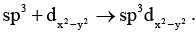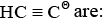Courses

# Test: Chemical Bonding- 2

## 30 Questions MCQ Test Inorganic Chemistry | Test: Chemical Bonding- 2

Description
This mock test of Test: Chemical Bonding- 2 for Chemistry helps you for every Chemistry entrance exam. This contains 30 Multiple Choice Questions for Chemistry Test: Chemical Bonding- 2 (mcq) to study with solutions a complete question bank. The solved questions answers in this Test: Chemical Bonding- 2 quiz give you a good mix of easy questions and tough questions. Chemistry students definitely take this Test: Chemical Bonding- 2 exercise for a better result in the exam. You can find other Test: Chemical Bonding- 2 extra questions, long questions & short questions for Chemistry on EduRev as well by searching above.
QUESTION: 1

Solution:
QUESTION: 2

Solution:
QUESTION: 3

### Which of the following set of ions/ molecules is isoelectric and structural?

Solution:
QUESTION: 4

In allene (C3H4), the type(s) of hybridisation of the carbon atoms is (are)

Solution:
QUESTION: 5

The atom that form discrete polyatomic molecule in its elemental state are:

Solution:

Sulfur forms discrete polyatomic molecule as in S8

Carbon forms discrete polyatomic molecules as in diamond, etc.

QUESTION: 6

Which one of the following pairs is isoelectronic?

Solution:
QUESTION: 7

The structure of XeF4 is:

Solution:
QUESTION: 8

Which of the following species has two non bonded electron pairs on the central atom:

Solution:

In TeCl4 out of 6 electrons:bonded electrons are 4,non-bonded electron pair is 1.
In ClF3 out of 7 electrons:bonded electrons are 3,non-bonded electron pairs are 2.
In PCl3 out of 5 electrons:bonded electrons are 3,non-bonded electron pair is 1.
In ICl2− out of (7+1=8) electrons:bonded electrons are 2,non-bonded electron pairs are 3.

QUESTION: 9

The shape and expected hybridization of BrO3- and HOCl are:

Solution:
QUESTION: 10

I3- ion is linear having the hybridization:

Solution:
QUESTION: 11

The hybridization of atomic orbital of nitrogen in NO2+, NO3- and NH4+ are:

Solution:
QUESTION: 12

Among the molecular ions, the dπ - pπ bond is possible in:

Solution:
QUESTION: 13Now the shape becomes:

Solution:
QUESTION: 14

Angles in XeO3F2 are equal with which molecule:

Solution:
QUESTION: 15

Hybridization in Boron in BF3-ether mixture is:

Solution:
QUESTION: 16

Hybridization on anionic carbon in allyl anion is:

Solution:
QUESTION: 17

Hybridization and shape of N(SiH3)3 is:

Solution:
QUESTION: 18

Hybridization in carbon atoms ofSolution:
QUESTION: 19

Hybridization in (C6H5)3C (on negative carbon) is:

Solution:
QUESTION: 20

Hybridization of carbon C3O2 and CO2 are:

Solution:
QUESTION: 21

Hybridization on central atom in SOF4 is:

Solution:
QUESTION: 22

Hybridization on central atom in Be2C is:

Solution:
QUESTION: 23

Hybridization in N2 is:

Solution:

Pi bond electrons don’t contribute when we calculate the hybridization. So due to one sigma bond and one lone pair hybridization will be “sp”.

Hence D is correct.

QUESTION: 24

Hybridization on the central atom in H3Ois:

Solution:
QUESTION: 25

Hybridization in Si in Si4O116– is:

Solution:
QUESTION: 26

In octahedral structure the pair of ‘d’ orbitals invo lved is:

Solution:
QUESTION: 27

The ONO angle is maximum is:

Solution:
QUESTION: 28

Hybridization state of boron and oxygen in boric acid is:

Solution:
QUESTION: 29

Which is correct order of bond angle:

Solution:
QUESTION: 30

If CH4 is assumed to give two disubst ituted product. Then, the shape of CH4 will be:

Solution: